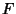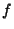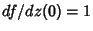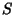## Bloch Constant

N.B. A detailed on-line essay by S. Finch was the starting point for this entry.

Letbe the set of Complex analytic functionsdefined on an open region containing the closure of the unit disksatisfyingand. For eachin, letbe the Supremum of all numberssuch that there is a diskinon whichis One-to-One and such thatcontains a disk of radius. In 1925, Bloch (Conway 1978) showed that. Define Bloch's constant byAhlfors and Grunsky (1937) derivedThey also conjectured that the upper limit is actually the value of,(Le Lionnais 1983).

References

Conway, J. B. Functions of One Complex Variable, 2nd ed. New York: Springer-Verlag, 1989.

Finch, S. Favorite Mathematical Constants.'' http://www.mathsoft.com/asolve/constant/bloch/bloch.html

Le Lionnais, F. Les nombres remarquables. Paris: Hermann, p. 25, 1983.

Minda, C. D. Bloch Constants.'' J. d'Analyse Math. 41, 54-84, 1982.